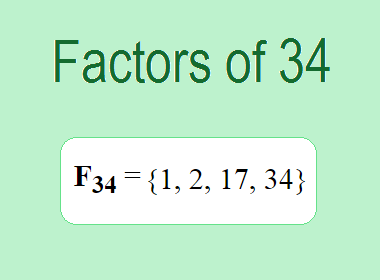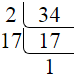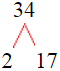# Factors of 34The factors of 34 are 1, 2, 17, and 34 i.e. F34 = {1, 2, 17, 34}. The factors of 34 are all the numbers that can divide 34 without leaving a remainder.

We can check if these numbers are factors of 34 by dividing 34 by each of them. If the result is a whole number, then the number is a factor of 34. Let's do this for each of the numbers listed above:

·        1 is a factor of 34 because 34 divided by 1 is 34.

·        2 is a factor of 34 because 34 divided by 2 is 17.

·        17 is a factor of 34 because 34 divided by 17 is 2.

·        34 is a factor of 34 because 34 divided by 34 is 1.

## How to Find Factors of 34?

1 and the number itself are the factors of every number. So, 1 and 34 are two factors of 34. To find the other factors of 34, we can start by dividing 34 by the numbers between 1 and 34. If we divide 34 by 2, we get a remainder of 0. Therefore, 2 is a factor of 34. If we divide 34 by 3, we get a remainder of 1. Therefore, 3 is not a factor of 34.

Next, we can check if 4 is a factor of 34. If we divide 34 by 4, we get a remainder of 2. Therefore, 4 is not a factor of 34. We can continue this process for all the possible factors of 34.

Through this process, we can find that the factors of 34 are 1, 2, 17, and 34. These are the only numbers that can divide 34 without leaving a remainder.

********************

********************

## Properties of the Factors of 34

The factors of 34 have some interesting properties. One of the properties is that the sum of the factors of 34 is equal to 54. We can see this by adding all the factors of 34 together:

1 + 2 + 17 + 34 = 54

Another property of the factors of 34 is that the prime factors of 34 are 2 and 17.

## Applications of the Factors of 34

The factors of 34 have several applications in mathematics. One of the applications is in finding the highest common factor (HCF) of two or more numbers. The HCF is the largest factor that two or more numbers have in common. For example, to find the HCF of 34 and 51, we need to find the factors of both numbers and identify the largest factor they have in common. The factors of 34 are 1, 2, 17, and 34. The factors of 51 are 1, 3, 17, and 51. The largest factor that they have in common is 17. Therefore, the HCF of 34 and 51 is 17.

Another application of the factors of 34 is in prime factorization. Prime factorization is the process of expressing a number as the product of its prime factors. The prime factors of 34 are 2 and 17, since these are the only prime numbers that can divide 34 without leaving a remainder. Therefore, we can express 34 as:

34 = 2 × 17

We can do prime factorization by division and factor tree method also. Here is the prime factorization of 34 by division method,34 = 2 × 17

Here is the prime factorization of 34 by the factor tree method,34 = 2 × 17

## Conclusion

The factors of 34 are the numbers that can divide 34 without leaving a remainder. The factors of 34 are 1, 2, 17, and 34. The factors of 34 have some interesting properties, such as having a sum of 54. The factors of 34 have several applications in mathematics, such as finding the highest common factor and prime factorization.